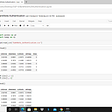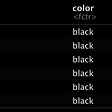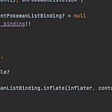# Statistical functions and Statistical Distributions

## Mean

The mean is the sum of all values divided by the number of values.

import statistics as stx = [4,5,1,2,7,2,6,9,3,7,2]print('Mean:', st.mean(x))Mean: 4.363636363636363

##1) ✍ Implement mean from scratch.

def mean(X):    sum = 0    for i, n in enumerate(X):        sum += X[i]     return sum/(i + 1)print('Mean:', mean(x))Mean: 4.363636363636363x[4, 5, 1, 2, 7, 2, 6, 9, 3, 7, 2]sum = 0sum(x)48

## Median

The median is the middle value in the list of numbers.

print('Median:', st.median(x))Median: 4

##2) ✍ Implement median from scratch.

def median(X):    N = len(X)   #0 1 2 3 4 5 6 7    #X.sort()    X = sorted(X)    if N%2 != 0:        return X[N//2]    else:        return round((X[N//2] + X[N//2 - 1])/2, 1)print('Median:', median(x))Median: 4

## Mode

The mode is the value that occurs most often. If no number in the list is repeated, then there is no mode for the list.

x = [1,2,3,3,5]print(x)print('Mode:', st.mode(x))[1, 2, 3, 3, 5]Mode: 3

## ✍ 3) Implement mode from scratch.

#mode = max(set(x), key=x.count)def mode(X):    x = [(i, X.count(i)) for i in X]    y = list(set(x))          y.sort(key=lambda y: y, reverse=True)    y.sort(key=lambda y: y, reverse=True)      return(y)x = [1, 2, 2, 3]print('Mode:', mode(x))Mode: 2X = [2,2,2,3,3,5,5,5,5,8,8]X.sort()count = 1count_number = Xcount_max = 1for i in range(1,len(X)):  if X[i] == X[i-1]:    count = count + 1    if count > count_max:      count_max = count      count_number = X[i]  else:    count = 1print(count_number)5from collections import CounterCounter(X).most_common()5l = [2,5,4,8,3,8,6,8,6]s = list(set(l))c = []for i in s:    c.append(l.count(i))d = {k:v for k, v in zip(s,c)}  for k in d.keys():    if(d[k] == max(c)):        print(k)8

## Range

Range is the differene between the largest data value and the smallest data value in the set.

import numpy as npx = [1, 4, 8]print(x)print('Range:', np.ptp(x))[1, 4, 8]Range: 7

##4) ✍ Implement range from scratch.

np.max(x) - np.min(x)7def my_range(l):    l_min, l_max = float("inf"), -float("inf")    for elem in l:        l_min = min(l_min, elem)        l_max = max(l_max, elem)          return l_max - l_minmy_range([1,2,4,3])3

## Interquartile Range (IQR)

The interquartile range (IQR), also called midspread, is the length of the middle 50% of data interval. The IQR is calculated as the difference between the third or upper quartile ($Q_3$) and the first or lower quartile ($Q_1$).

import numpy as npfrom scipy.stats import iqr#x = [4,5,1,2,7,2,6,9,3,7,2]x = [1, 4, 5, 6, 6, 7, 11, 12, 15, 17]print('IQR of data:', iqr(x, ))IQR of data: 6.5q3, q1 = np.percentile(x, [75 , 25], interpolation = "nearest")print('IQR of data:', q3 - q1)IQR of data: 7print(q1,q3)5 12

##5) ✍ Implement IQR from scratch.

def median(X, N):        X = sorted(X)    if N%2 != 0:        return X[N//2]    else:        return (X[N//2] + X[N//2 - 1])/2.def Interquartile_Range(X):    N = len(X)        X.sort()        Q1 = median(X[:N//2], N//2)    print(Q1)        if N%2 == 0:        Q3 = median(X[N//2:], N//2)     else:        Q3 = median(X[N//2+1:], N//2)     print(Q3)        return (Q3 - Q1)x = [1, 4, 5, 6, 6, 7, 11, 12, 15, 17]print("%0.1f" % Interquartile_Range(x))5127.0

## Variance

Variance is the expectation of the squared deviation of a random variable from its mean. Informally, it measures how far a set of (random) numbers are spread out from their average value.

print(x)print('Population variance:', st.pvariance(x))print('Sample variance:', st.variance(x))[1, 4, 5, 6, 6, 7, 11, 12, 15, 17]Population variance: 23.64Sample variance: 26.266666666666666

## Standard Deviation

The standard deviation is the square root of its variance.

?np.stdprint('Population standard deviation:', st.pstdev(x))print('Sample standard deviation:', st.stdev(x))Population standard deviation: 4.862098312457287Sample standard deviation: 5.125101625008686print('Population standard deviation:',np.std(x, ddof=0)) # ddof: deduct degrees of freedomprint('Sample standard deviation:', np.std(x, ddof=1))Population standard deviation: 4.862098312457287Sample standard deviation: 5.125101625008686

## 6) ✍ Implement population standard deviation from scratch.

def mean(X, N):    sum = 0    for i in range(len(X)):        sum += X[i]     return sum/N def standard_deviation(X):    N = len(X)        mu = mean(X, N)      tmp = 0    for i in range(N):        tmp += (X[i] - mu)**2        return (tmp/N)**0.5x = [1,2,3,4,5,6]standard_deviation(x)1.707825127659933

## Skewness

Skewness [Ref] is a measure of symmetry, or more precisely, the lack of symmetry. A distribution, or data set, is symmetric if it looks the same to the left and right of the center point.

• The skewness for a normal distribution is zero, and any symmetric data should have a skewness near zero.
• Negative values for the skewness indicate data that are skewed left and positive values for the skewness indicate data that are skewed right. By skewed left, we mean that the left tail is long relative to the right tail.
• Similarly, skewed right means that the right tail is long relative to the left tail.
• If the data are multi-modal, then this may affect the sign of the skewness.
from scipy.stats import skewimport scipy.stats as st#data = np.random.normal(0, 1, 1000000)data = np.random.uniform(0, 1, 100000)print("skewness:",st.skew(data))skewness: -0.0030195334962366182

## Kurtosis

Kurtosis is a measure of whether the data are heavy-tailed or light-tailed relative to a normal distribution. That is, data sets with high kurtosis tend to have heavy tails, or outliers. Data sets with low kurtosis tend to have light tails, or lack of outliers. A uniform distribution would be the extreme case.

from scipy.stats import kurtosisprint("kurtosis:", kurtosis(data))kurtosis: -1.198085296060503

## Discrete uniform distribution

The discrete uniform distribution is a symmetric probability distribution whereby a finite number of values are equally likely to be observed. Its probability mass function is $$p(x) = \frac{1}{b — a}$$ for $x = a, …, b — 1$.

Python syntax:

scipy.stats.randint(low, high+1)

np.random.randint(low, high+1)

from scipy.stats import randintlow = 3high = 8X = randint(low, high + 1) # Declare X to be a uniform random variableX.rvs(size= 10) # Get 10 random samples form Xarray([8, 6, 7, 6, 6, 5, 4, 3, 5, 7])?sns.distplotimport seaborn as snsimport matplotlib.pyplot as plt%matplotlib inlinedef plot_dist(X, title):    ax = sns.distplot(X, kde=False, color='crimson', hist_kws={"linewidth": 15,'alpha':1})    ax.set(xlabel= 'x', ylabel='Frequency', title = title);X = randint.rvs(low, high + 1, size= 10000)print("skewness:",skew(X))print("kurtosis:", kurtosis(X))plot_dist(X, 'Uniform Distribution')skewness: -0.019059873221003085kurtosis: -1.2545266059524007/usr/local/lib/python3.6/dist-packages/seaborn/distributions.py:2551: FutureWarning: distplot is a deprecated function and will be removed in a future version. Please adapt your code to use either displot (a figure-level function with similar flexibility) or histplot (an axes-level function for histograms).  warnings.warn(msg, FutureWarning)

## Bernoulli distribution

A Bernoulli trial is an experiment that has two outcomes that can be encoded as success ($x = 1$) or failure ($x = 0$). The Bernoulli Probability Mass Function (PMF) is $$p(x) = {p^x}{(1 — p)^{(1-x)}}$$ where $x \in {0, 1}$.

Python syntax:

scipy.stats.bernoulli(theta)

np.random.choice([0, 1], p=[1-theta, theta])

from scipy.stats import bernoullip = 0.25         # probability of successX = bernoulli(p) # Declare X to be a bernoulli random variableX.rvs(10)        # Get 10 random samples form Xarray([0, 0, 0, 0, 1, 1, 0, 0, 0, 0])print('P(X = 0) =', X.pmf(0))print('P(X = 1) =', X.pmf(1))print('P(X <= 0.5) =', X.cdf(0.5)) # P(X<= 0.5) = P(X = 0) = .75print('E[X] =', X.mean())print('Var(X) =', X.var())print('Std(X) =', X.std())P(X = 0) = 0.75P(X = 1) = 0.25P(X <= 0.5) = 0.75E[X] = 0.25Var(X) = 0.1875Std(X) = 0.4330127018922193import seaborn as snsX = bernoulli.rvs(p= 0.6, size= 1000)print("skewness:",skew(X))print("kurtosis:", kurtosis(X))plot_dist(X, 'Bernoulli Distribution')skewness: -0.37860678521640406kurtosis: -1.8566569021880999/usr/local/lib/python3.6/dist-packages/seaborn/distributions.py:2551: FutureWarning: distplot is a deprecated function and will be removed in a future version. Please adapt your code to use either displot (a figure-level function with similar flexibility) or histplot (an axes-level function for histograms).  warnings.warn(msg, FutureWarning)

## Binomial Distribution

The binomial distribution is used to obtain the probability of observing $x$ successes in $n$ trials, with the probability of success on a single trial denoted by $p$. The binomial distribution assumes that $p$ is fixed for all trials. The formula for the binomial probability mass function is $$p(x) = {n\choose x} {p^x}{(1 — p)^{(n-x)}}$$ where $x \in {0, 1,…, n}$, and ${n\choose x}$ is the binomial coefficient that calculates different ways of distributing $x$ successes in a sequence of $n$ trials.

Python syntax:

scipy.stats.binom(N, theta)

np.random.binomial(N, theta)

from scipy.stats import binomn = 7    # Number of trials (number of coins)p = 0.5  # Probability of success in each trial sample_size = 5 # Sample size (number of experiments)X = binom.rvs(n = n, p = p, size = sample_size)Xarray([6, 4, 2, 3, 2])x = 2 # Number of successes soughtX = binom(n = n, p = p)             print('P(X = %d) = %f' %(x, X.pmf(x)))print('P(X <= %d) = %f' % (x, X.cdf(x)))print('E[X] =', X.mean())print('Var(X) =', X.var())print('Std(X) =', X.std())X = binom.rvs(n = 10, p = 0.3, size= int(1e6))print("skewness:",skew(X))print("kurtosis:", kurtosis(X))plot_dist(X, 'Binomial Distribution')

## Geometric distribution

We perform a series of Bernoulli trials with probability of success $p$ until we get a success. The probability that the $x$-th trial (out of $x$ trials) is the first success is $$p(x) = {(1 — p)^{(x-1)}}{p}$$ where $x \ge 1$.

Python syntax:

scipy.stats.geom(theta)

np.random.geometric(theta)

from scipy.stats import geomp = 0.2 # probability of success on each trialX = geom.rvs(p, size= 1000)print("skewness:",skew(X))print("kurtosis:", kurtosis(X))plot_dist(X, 'Geometric Distribution')

## Poisson distribution

The Poisson distribution is a discrete probability distribution that expresses the probability of a given number of events occurring in a fixed interval of time or space if these events occur with a known constant rate and independently of the time since the last event. The Poisson probability that exactly $x$ successes occur in an interval, with the mean number of successes $\lambda$, is $$p(x) = exp(-\lambda)\frac{{{\lambda}^x}}{{x!}}$$ where $x \ge 1$.

Python syntax:

scipy.stats.poisson(lam)

np.random.poisson(lam)

from scipy.stats import poissonlam = 3 # average number of events per intervalX = poisson.rvs(lam, size= 10000)print("skewness:",skew(X))print("kurtosis:", kurtosis(X))plot_dist(X, 'Poisson Distribution')

## Uniform distribution

Any outcome in a given range has equal probability.

Python syntax:

scipy.stats.uniform(low, high)

np.random.uniform(low, high)

from scipy.stats import uniformlow = 3high = 8X = uniform.rvs(low, high - low, size= 10000)print("skewness:",skew(X))print("kurtosis:", kurtosis(X))plot_dist(X, 'Uniform Distribution')

## Normal Distribution

The probability density of the normal (Gaussian) distribution is $$f(x) = \frac{1}{\sqrt{2\pi{{\sigma}²}}} exp(- \frac{(x — \mu)²}{2{\sigma}²})$$ where $\mu$ is the mean, and ${\sigma}²$ is the variance.

Python syntax:

scipy.stats.norm(mu, sigma)

np.random.normal(mu, sigma)

from scipy.stats import normprint(1e6)X = norm.rvs(loc=0, scale=1, size=int(1e6))print("skewness:",skew(X))print("kurtosis:", kurtosis(X))plot_dist(X, 'Normal Distribution')

## Student’s t distribution

The Student’s t-distribution (or simply the t-distribution) is given by $$f(x) = \frac{\Gamma(\frac{v+1}{2})}{\sqrt{\pi v} \Gamma(v)} (1 + \frac{x²}{v})^{-\frac{v+1}{2}}$$ where $x$ is a real number, $Î½ > 0$ is the degrees of freedom parameter, and $\Gamma$ is the gamma function.

Python syntax:

scipy.stats.t(nu, mu, sigma)

mu + sigma * np.random.standard_t(nu)

from scipy.stats import tdf = 3X = t.rvs(df, size=1000)print("skewness:",skew(X))print("kurtosis:", kurtosis(X))plot_dist(X, 't-distribution')

## Chi-square distribution

The chi-square distribution with $k$ degrees of freedom is the distribution of a sum of the squares of $k$ independent standard normal random variables. Its PDF is $$f = \frac{1}{2^{\frac{k}{2}} \Gamma(\frac{k}{2})} x^{\frac{k}{2} — 1} exp(-\frac{x}{2})$$ where $x>0$ and $k>0$.

Python syntax:

scipy.stats.chi2(nu)

np.random.chisquare(nu)

from scipy.stats import chi2df = 55X = chi2.rvs(df, size=1000)print("skewness:",skew(X))print("kurtosis:", kurtosis(X))plot_dist(X, 'Chi-square Distribution')

## Exponential distribution

The exponential distribution is the probability distribution of the time between events in a Poisson process. The PDF of an exponential distribution is $$f = \lambda exp(-\lambda x)$$ where $x \ge 0$ and $\lambda > 0$ is the parameter of the distribution, often called the rate parameter.

Python syntax:

scipy.stats.expon(loc=0, scale=1/lambda)

np.random.exponential(1/lambda)

from scipy.stats import exponX = expon.rvs(loc=0, scale=1, size=1000)print("skewness:",skew(X))print("kurtosis:", kurtosis(X))plot_dist(X, 'Exponential Distribution')

## Law of Large Numbers

The law of large numbers (LLN) states that, the average of the results obtained from a large number of trials should be close to the expected value, and will tend to become closer as more trials are performed.

Let ${X_1, X_2, …, X_n}$ be a random sample of size $n$ drawn iid from a distribution with mean $\mu$. According to LLN, we have

$$\bar{X} = \frac{X_1 + X_2 + …+ X_n}{n} \to \mu$$ for large $n$.

For example, a single roll of a fair, six-sided dice produces one of the numbers 1, 2, 3, 4, 5, or 6, each with equal probability. Therefore, the expected value of the average of the rolls is: $$\frac{1+2+3+4+5+6}{6}=3.5$$ According to the law of large numbers, if a large number of six-sided dice are rolled, the average of their values (the sample mean) is likely to be close to 3.5 (the population mean), with the precision increasing as more dice are rolled [Ref]. In the following, we have an illustration of the law of large numbers using a particular run of rolls of a single dice.

np.random.seed(123)N = 1000 # sample sizerolls = np.random.randint(1, 7, N)sample_means = []for i in range(N):    sample_means.append(rolls[:i + 1].mean())    plt.plot(range(1, N+1), 3.5*np.ones(N), 'r', label = 'Population mean')plt.plot(range(1, N+1), sample_means, 'b', label = 'Sample mean')plt.xlabel('Sample size (N)')plt.ylabel("Mean")plt.legend()plt.show()#rolls

## 7) ✍ Illustrate LLN for samples drawn iid from a normal distribution with mean of 50 and a variance of 10.

?np.random.normalfrom scipy.stats import normimport numpy as npimport matplotlib.pyplot as pltX = norm(loc= 50, scale= np.sqrt(10))mu = X.mean()var = X.var()print('Population mean', mu)print('Population variance', var)N = 1000 # sample sizex = X.rvs(size = N)#, random_state= 123)sample_means = []for i in range(N):    sample_means.append(sum(x[:i + 1])/(i+1))    plt.plot(range(1, N+1), mu*np.ones(N), 'r', label = 'Population mean')plt.plot(range(1, N+1), sample_means, 'b', label = 'Sample mean')plt.xlabel('Sample size (N)')plt.ylabel("Mean")plt.legend(loc = 'lower right')plt.show()Population mean 50.0Population variance 10.000000000000002

## Central Limit Theorem

The central limit theorem (CLT) dictates that the distribution of sample means (calculated across different random samples from our overall population) will be normally distributed. Mathematically, let ${X_1, X_2, …, X_n}$ be a random sample of size $n$ drawn iid from a distribution with mean $\mu$ and variance ${\sigma}²$, and define the sample average of the random variables as $$\bar{X} = \frac{X_1 + X_2 + … + X_n}{n}$$ The CLT states that $$\bar{X} \sim N(\mu, \frac{{\sigma}²}{n})$$ for large $n$.

## ✍ 8) Test CLT for iid Gamma random variables using scipy.

from scipy.stats import gamma, normimport numpy as npimport matplotlib.pyplot as plta, loc = 2, 3X = gamma(a, loc)mu = X.mean()var = X.var()print('Population mean:', mu) print('Population variance:', var) N = 50 # sample size   - corresponding to nnum_trials = 100000 # Number of trials - how many many we want to generatesamples = X.rvs(size = (num_trials, N))samples_means = samples.mean(axis= 1)print('Mean of all the sample means:', np.mean(samples_means))print('Variance of all the sample means =', np.var(samples_means))print('var/N:', var/N)plt.hist(samples_means, bins= 100, density=True)S_N = norm(loc= mu, scale= np.sqrt(var/N))x = np.linspace(S_N.ppf(0.001), S_N.ppf(0.999), 100)plt.plot(x, S_N.pdf(x), 'r')plt.show()Population mean: 5.0Population variance: 2.0Mean of all the sample means: 4.999268307684889Variance of all the sample means = 0.039875471278788675var/N: 0.04

## 9) ✍ Test CLT for iid uniform random variables in range of [5, 10] using numpy.

import numpy as npimport matplotlib.pyplot as plt%matplotlib inlineshape, scale = 2., 2.populaiton_size = 100000s = np.random.uniform(low= 5, high= 10, size = populaiton_size)mu = np.mean(s) # mu = (high + low)/2var = np.var(s) # var = (high - low)**2/12print('Population mean:', mu) print('Population variance:', var) plt.hist(s)plt.show()N = 20num_trials = 10000 # Number of trialssamples = [np.mean(np.random.choice(s, size = N)) for _ in range(num_trials)]print('Mean of all the sample means:', np.mean(samples))print('Variance of all the sample means:', np.var(samples))print('var/N:', var/N)plt.hist(samples, density=True)def normal_pdf(x, mu, sigma):    return (1/(sigma*np.sqrt(2*np.pi)) *np.exp( - (x - mu)**2 /(2*sigma**2)))x = np.linspace(6, 9, 100)plt.plot(x, normal_pdf(x, mu = mu, sigma = np.sqrt(var/N)), 'r')plt.show()Population mean: 7.5082694056875Population variance: 2.0802442142948188Mean of all the sample means: 7.506555371635119Variance of all the sample means: 0.1031419760228997var/N: 0.10401221071474094

--

--

--

## More from Mohamed Niang

Machine Learning Scientist

Love podcasts or audiobooks? Learn on the go with our new app.

## Local Hack Day: how I walked into my 1st Hackathon? Even better with 1st prize!!!!## Git Happens tryhackme## At Branch we process and store vast amounts of data; data generated from over 15 billion user…## Setting up a CI/CD pipeline in Azure DevOps for Blazor and Deploy to AWS## BANK NOTE AUTHENTICATION END TO END WITH DOCKERS## Create custom REST Resource for GET and POST method in Drupal 8## The Secret Ingredient | QA Entwined## Mohamed Niang

Machine Learning Scientist

## Automated Pathologists?## Working with a Deck of Cards in R## Basics of Sampling Theory## Data Binding